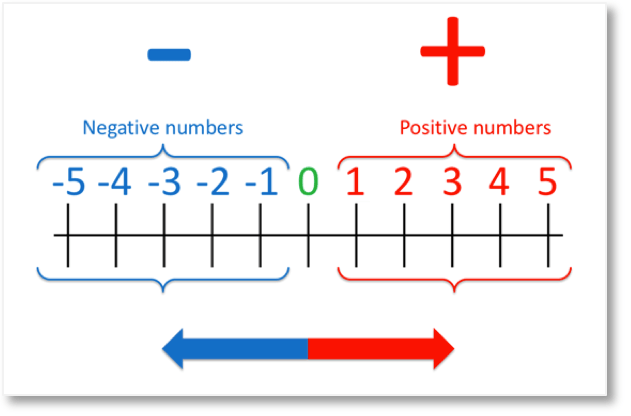# Roman Numerals to 100

Roman Numerals to 100• I is worth 1.
• V is worth 5.
• X is worth 10.
• L is worth 50.
• C is worth 100.
• We can create other numbers by adding these numerals.
• We repeat the numerals up to 3 times in a row from the largest value numerals to the smallest.
• 4 is written as 1 before 5: ‘IV’ and 9 is written as 1 before 10: ‘IX’.
• 40 is written as 10 before 50: ‘XL’ and 90 is written as 10 before 100: ‘XC’.
• We repeat numerals 1 to 9 (shown in red) for each set of ten.

The Roman numerals to 100 are made up of the numerals I (1), V (5), X (10), L (50) and C (100).

We can repeat them up to 3 times to add them.• To write 84 in Roman numerals we write the 80 and then add the 4.
• 80 is made by counting on from 50 with three tens.
• 80 = 50 + 10 + 10 + 10, which can be written as LXXX.
• 4 is written as 1 before 5: IV.
• 84 is written as LXXX plus IV which we write as LXXXIV.#### Roman Numerals to 100 Interactive Question Generator

Roman Numerals to 100: Interactive Questions

# Roman Numerals to 100

## What are the Roman Numerals to 100?

Here is a chart showing all of the roman numerals to 100.The roman numerals to 100 are written using the 5 numerals of I, V, X, L and C.

Each of these numerals has the following values:

• I = 1
• V = 5
• X = 10
• L = 50
• C = 100## Rules for Roman Numerals

The rules for Roman numerals are as follows: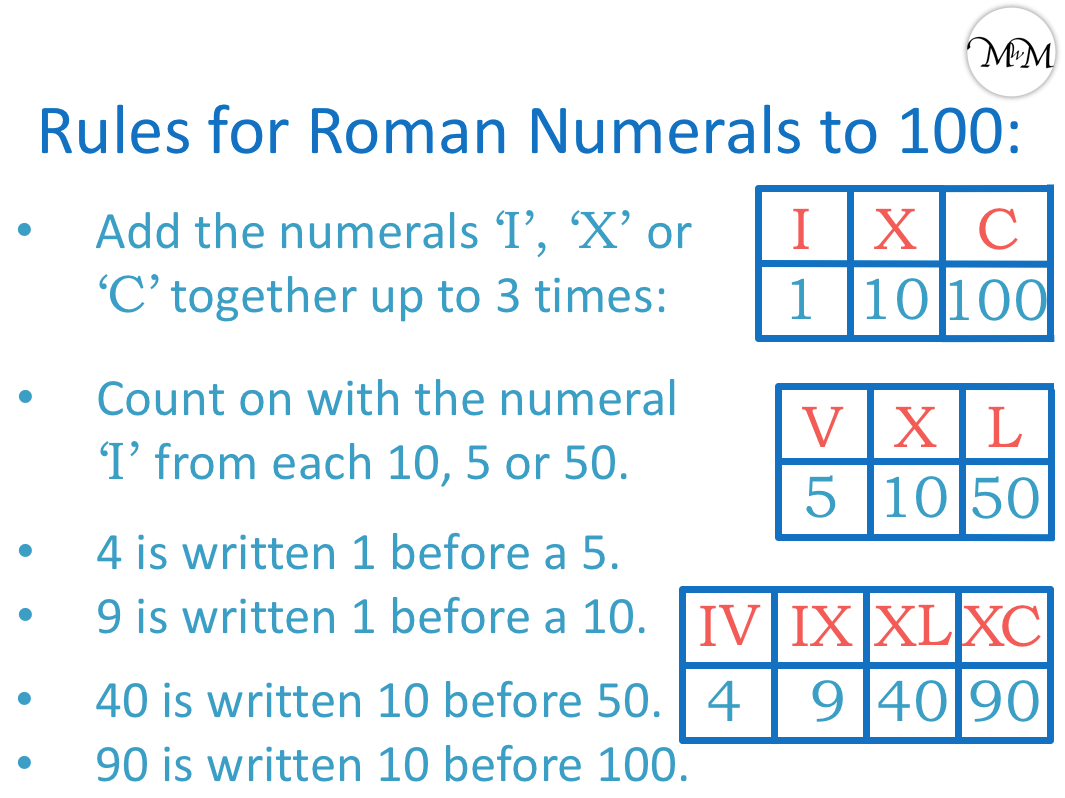• Repeat the numerals of I (1), X (10) or C (100) to add them up to 3 times in a row.
• If a numeral follows another numeral that is of the same value or larger, then add it.
• Count on using the numeral ‘I’ from each X (10), V (5) or L (50).
• If a numeral appears before a larger numeral then subtract it from the larger numeral.

There are only two digits that are created by subtracting. These are 4 and 9.

• 4 is written as 1 before 5: ‘IV’.
• 40 is written as 10 before 50: ‘XL’.
• 9 is written as 1 before 10: ‘IX’.
• 90 is written as 10 before 100: ‘XC’.

We can add the same numeral up to three times but not more than this.

For example, the numeral I can be written twice as II, which equals 2.

We can make 3 with three I numerals: III.

However we cannot write 4 as IIII because we have used the same numeral more than three times. Instead the digit 4 is one of our special digits that we write as a subtraction.

We subtract 1 from 5 to make 4. We write four as IV.

Similarly we can add to five to make 6, 7 and 8.

Six is VI, which is 5 + 1.

Seven is VII, which is 5 + 1 + 1.

Eight is VIII, which is 5 + 1 + 1 + 1. Eight requires three of the same numeral, I.

We cannot write 9 as VIIII because this requires four of the numeral I. We can only use three at once.

9 is our second special digit that is made by subtracting.

9 is 1 before 10, written as IX.

Once we know the rules for Roman numerals 1 to 10, we can continue to use these to make larger numerals.

## Writing Numbers to 100 in Roman Numerals

To write Roman numerals, partition the number into its tens and units. First write the numerals for the tens digit and then write the numerals for the units digit.

We can combine the numerals of I, V, X, L and C to create all numbers up to 100.For example, we can partition 11 into 10 + 1.

10 is X and 1 is I and so, 11 is written as XI. Ten plus one.

12 is 10 + 2. We write this as XII.

XII means X + I + I, which means 10 + 1 + 1.

Here are the Roman numerals from 1 to 30.We can see that once we know the Roman numerals from 1 to 10, we can create the numerals to 30 by writing them after the appropriate tens digit numeral.

To write the numbers 11 to 20 in Roman numerals, write the Roman numerals 1 to 10 after the numeral ‘X’.

For example, 18 is made from 10 + 8.

We can write the numeral X for 10 and then follow this with VIII, which is 8.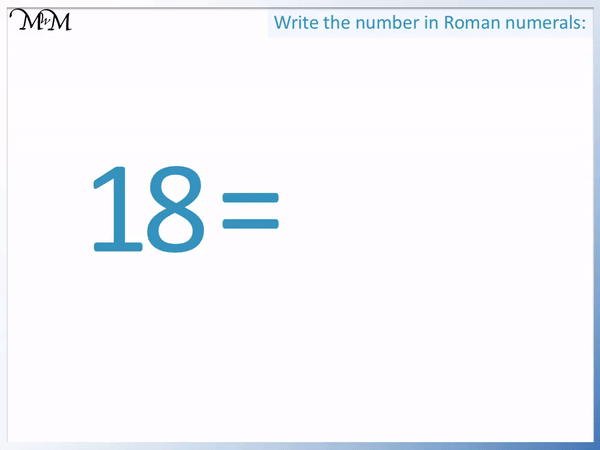18 is written as XVIII, which means 10 + 5 + 1 + 1 + 1.

Our numerals decrease in size from 10 to 5 to 1, so we add them. We have stuck to the rule of only using three of the same numeral at once.

To write the Roman numerals 21 to 30, we write the Roman numerals 1 to 10 after two X numerals.

XX is worth 10 + 10, which is 20. We write XX for twenty and then add on the Roman numerals between 1 and 10 afterwards to make the numbers from 21 to 30.

For example, 21 is partitioned into 20 + 1.

20 is two tens, written as XX.

We need to add 1 more to 20 to make 21.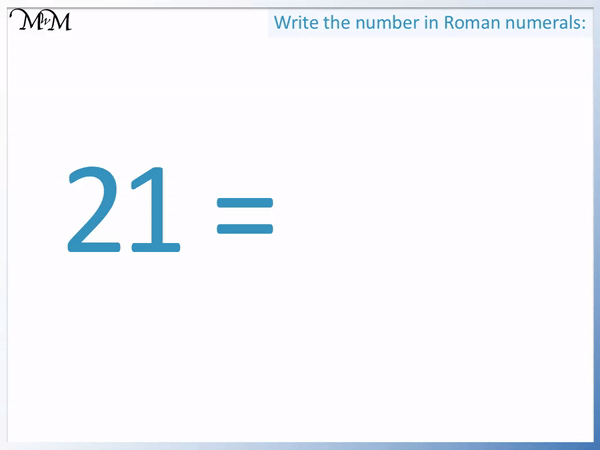21 is written as XXI, which means 10 + 10 + 1.

We can see in the list of Roman numerals to 30 below, that the numerals 1 to 9 are shown in red. There is a clear pattern of these numerals repeating from column to column. We simply put an extra X numeral in front every time that we move to the next column and add ten.For example, we can start with 5, move right one column to get to 15 and then move right one more column to get to 25.

We start at V for five. We add ten by writing an X numeral in front. XV is 15. Another X is written in front to make XXV, 25.

We can continue this pattern as we look at the Roman numerals 31 to 60.In the first column, we have the numerals to 40.

We have the Roman numerals 1 to 9 following three X numerals.

XXX is 30, which means 10 + 10 + 10. It is okay in Roman numerals to use the same numeral up to three times.

38 is made by partitioning 38 into 30 + 8.

We know that 30 is XXX and 8 is VIII.38 is written as XXXVIII, which means 10 + 10 + 10 + 5 + 1 + 1 + 1.

We cannot write 40 as four tens. XXXX is using four of the same Roman numeral, which is not allowed.

Like the number 4 is written as IV, 1 before 5, we need to write 40 as 10 before 50.

10 before 50 is XL. X is 10 and L is 50. For example, 43 is made of 40 + 3.

40 is XL and then 3 is III.43 is written as XLIII in Roman numerals.

XLIII is XL + III, which is 40 + 3.

Be careful not to think of XLIII as 10 + 50 + 1 + 1 + 1.

X (10) is a smaller value than L (50) and we do not write smaller numerals before larger numerals to add them. If a smaller numeral is written before a larger numeral, then subtract the smaller numeral from the larger numeral.

XL is 50 – 10, which is 40.

It is easiest to remember that 4, 40, 9 and 90 are created in this way. Both 4 and 9 are one less than the multiples of five, 5 and 10.

We can see all numbers in the 40 column start with XL.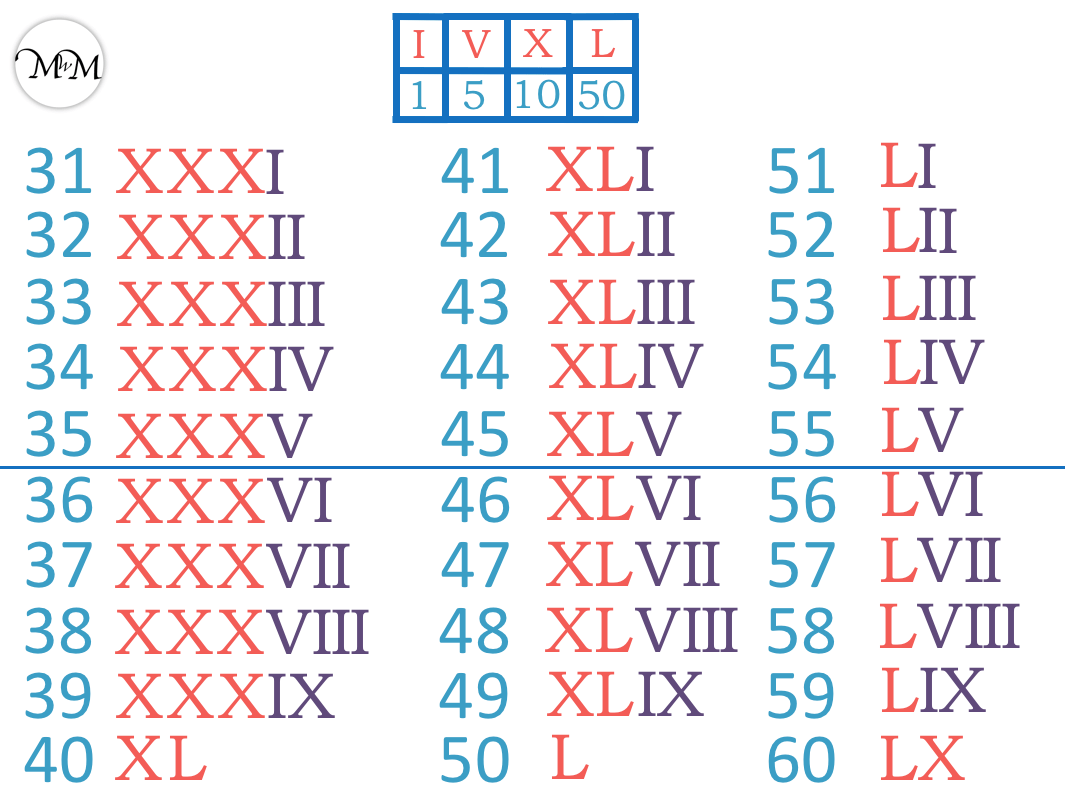50 is written as L in Roman numerals. 50 has its own Roman numeral just like 5 does.

All numbers in the fifties start with L and are followed by the Roman numerals 1 to 9.

60 is made by 50 + 10, written as LX.For example, 69 is written as 60 + 9.

60 is LX and 9 is IX.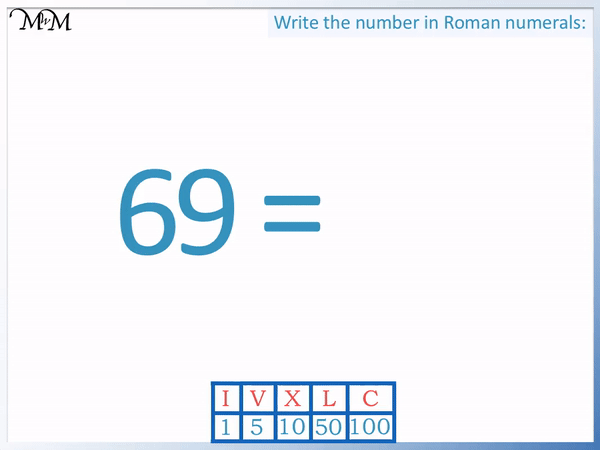69 is written as LXIX in Roman numerals. LX + IX means 60 + 9.

We can continue to count on from 50 to make 70 and 80.

70 is two tens more than 50, which is written as LXX. LXX means 50 + 10 + 10.

80 is three tens more than 50, which is LXXX. LXXX means 50 + 10 + 10 + 10.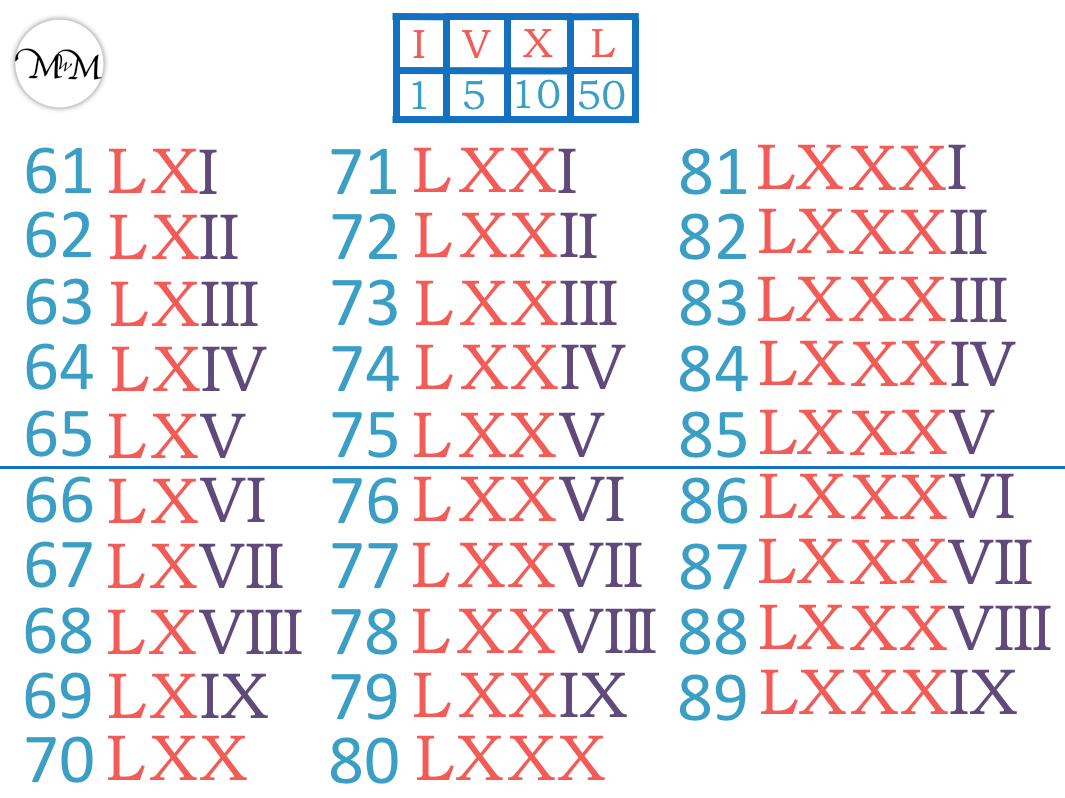For example, 84 is written as 80 + 4.

80 is LXXX, 50 + 10 + 10 + 10.

4 is IV, 1 before 5.84 is written as LXXXIV, which means L + X + X + X + IV.

Remember to look out for the special digits of 4 and 9, which are created by subtracting 1 from 5 or 10 respectively.

To write any larger numbers, we need our next Roman numeral.

100 is written as C in Roman numerals.We cannot write 90 as LXXXX because this uses four of the same numeral, X.

Like 9 is written as 1 before 10, 90 s written as 10 before 100.

90 is written as XC in Roman numerals. XC is 10 before 100. XC means 100 – 10.

We subtract 10 from 100, because when we have a smaller numeral in front of a larger numeral, we subtract the smaller numeral from the larger numeral.

All of the numbers in the nineties are written beginning with XC.For example, 92 can be partitioned into 90 + 2.

90 is XC and 2 is II.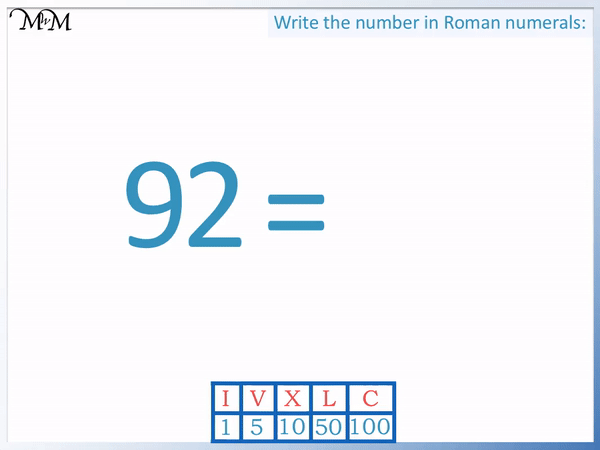92 is written as XCII in Roman numerals.

XCII means XC + II, 90 plus 2.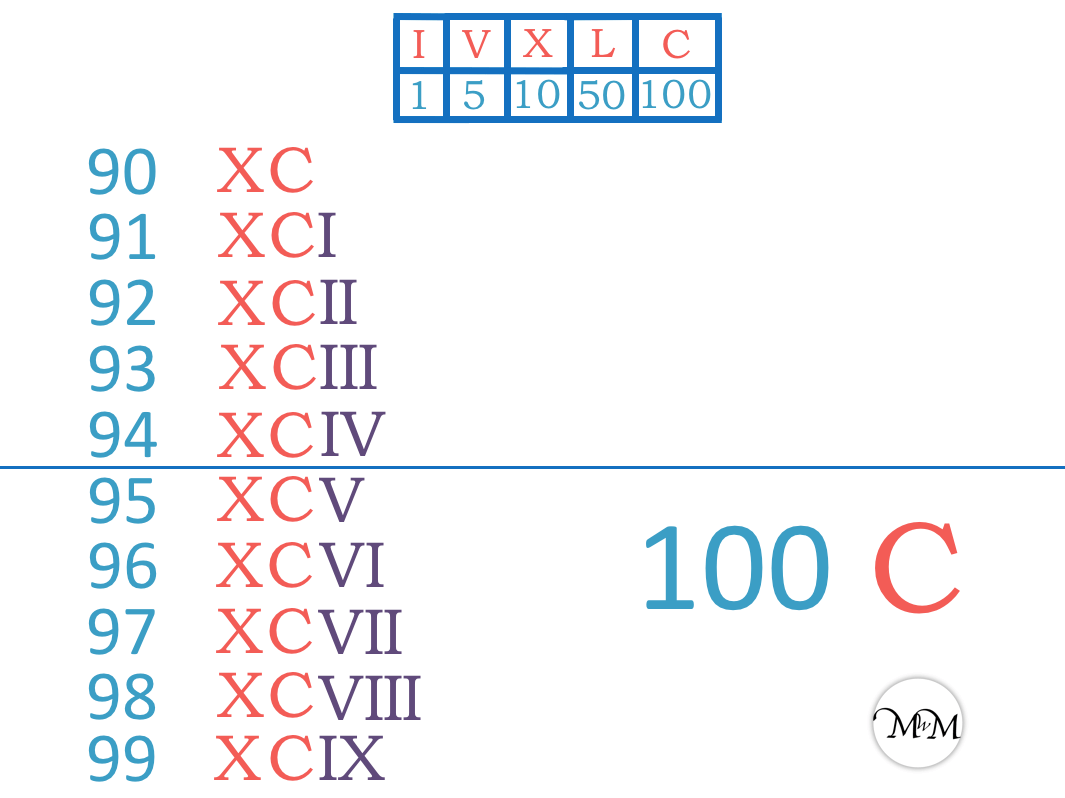We still continue to use the Roman numerals 1 to 9 with each new column.

## Where are Roman Numerals used Today?

Roman numerals are most commonly seen today on clock or watch faces. The numerals to 12 make up the 12 hours on the clock face. The digit 4 is often represented as ‘IIII’ on a clock face, instead of the usual ‘IV’. This is to distinguish it more clearly from other numerals such as VI for 6.

Roman numerals are often used in modern times to number more formal or significant events.

For example, some major events are numbered with Roman numerals, such as the World Wars I and II, along with the Olympic games.

Kings, queens and popes are often numbered with Roman numerals, such as Queen Elizabeth II and King Henry VIII. Some people named after others in their family may use these numerals too.

Old buildings and monuments often have significant dates carved on them in Roman numerals.

Book volumes or chapters, along with movie copyrights often use Roman numerals as it can appear more formal.

Roman numerals are not used frequently today primarily because they take up too much space and are difficult to use in mathematical calculations.

For example, 88 is much shorter than LXXXVIII.

Also by using our number system separately to the letters of the alphabet, it is easier to identify which is a number and which is a letter. Algebra is a branch of Mathematics that uses the letters of the alphabet and this would be very difficult if we still used Roman numerals today.Now try our lesson on Negative Numbers on a Number Line where we learn about negative numbers.